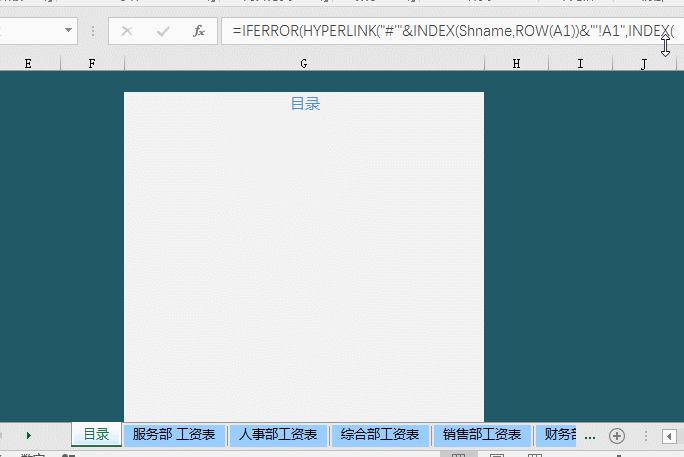01.取绝对值

=ABS(数字)

02.数字取整

=INT(数字)

03.数字四舍五入

=ROUND(数字,小数位数)

04.把公式返回的错误值显示为空

=IFERROR(A2/B2,””)

05.IF的多条件判断

=IF(AND(A2<500,B2=”未到期”),”补款”,””)

06.统计两表重复

=COUNTIF(Sheet15!A:A,A2)07.统计年龄在30~40之间的员工个数

=FREQUENCY(D2:D8,{40,29})

08.统计不重复的总人数

=SUMPRODUCT(1/COUNTIF(A2:A8,A2:A8))

09.按多条件统计平均值

F2公式

=AVERAGEIFS(D:D,B:B,”财务”,C:C,”大专”)

10.中国式排名公式

=SUMPRODUCT((\$D\$4:\$D\$9>=D4)*(1/COUNTIF(D\$4:D\$9,D\$4:D\$9)))11.隔列求和

=SUMIF(\$A\$2:\$G\$2,H\$2,A3:G3)

=SUMPRODUCT((MOD(COLUMN(B3:G3),2)=0)*B3:G3)12.单条件求和

=SUMIF(A:A,E2,C:C)

13.单条件模糊求和

14.多条求模糊求和

=SUMIFS(C2:C7,A2:A7,A11&”*”,B2:B7,B11)

15.多表相同位置求和

=SUM(Sheet1:Sheet19!B2)

16.按日期和产品求和

=SUMPRODUCT((MONTH(\$A\$2:\$A\$25)=F\$1)*(\$B\$2:\$B\$25=\$E2)*\$C\$2:\$C\$25)

17.单条件查找

=VLOOKUP(B11,B3:F7,4,FALSE)

18.双向查找

=INDEX(C3:H7,MATCH(B10,B3:B7,0),MATCH(C10,C2:H2,0))

19.查找最后一个符合条件记录20.多条件查找21.指定非空区域最后一个值查找

22.区间取值

## 六、字符串处理公式

23.多单元格字符合并

=PHONETIC(A2:A7)

24.截取除后3位之外的部分

=LEFT(D1,LEN(D1)-3)25.截取 – 之前的部分

=Left(A1,FIND(“-“,A1)-1)26.截取字符串中任一段

=TRIM(MID(SUBSTITUTE(\$A1,” “,REPT(” “,20)),20,20))27.字符串查找

=IF(COUNT(FIND(“河南”,A2))=0,”否”,”是”)

28.字符串查找一对多

=IF(COUNT(FIND({“辽宁”,”黑龙江”,”吉林”},A2))=0,”其他”,”东北”)

29.两日期间隔的年、月、日计算

A1是开始日期（2011-12-1），B1是结束日期(2013-6-10)。计算：

datedif函数第3个参数说明：

“Y” 时间段中的整年数。

“M” 时间段中的整月数。

“D” 时间段中的天数。

“MD” 天数的差。忽略日期中的月和年。

“YM” 月数的差。忽略日期中的日和年。

“YD” 天数的差。忽略日期中的年。

30.扣除周末的工作日天数

=NETWORKDAYS.INTL(IF(B2<DATE(2015,1,1),DATE(2015,1,1),B2),DATE(2015,1,31),11)31.创建工作表目录的公式

=MID(GET.WORKBOOK(1),FIND(“]”,GET.WORKBOOK(1))+1,99)&T(NOW())32.中英文互译公式

=FILTERXML(WEBSERVICE(“http://fanyi.youdao.com/translate?&i=”&A2&”&doctype=xml&version”),”//translation”)